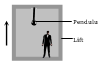• Subject:

...

• Topic:

...

­­A man measures the period of a simple pendulum inside a stationary lift and finds it to be T sec. If the lift accelerates upwards with an acceleration $\mathrm{g}}{4}$ , then the period of the pendulum will be(a) T

(b) $\frac{\mathrm{T}}{4}$

(c) $\frac{2\mathrm{T}}{\sqrt{5}}$

(d) $2\mathrm{T}\sqrt{5}$